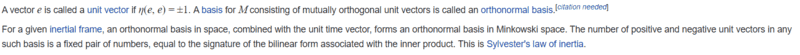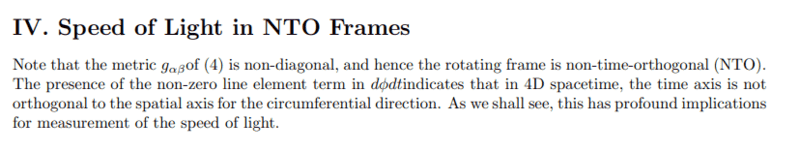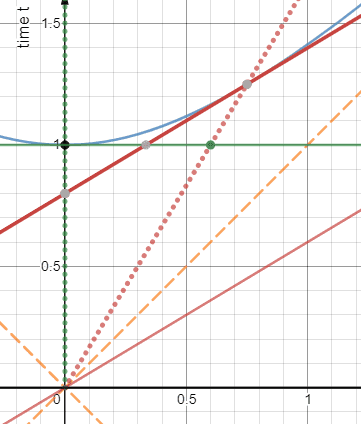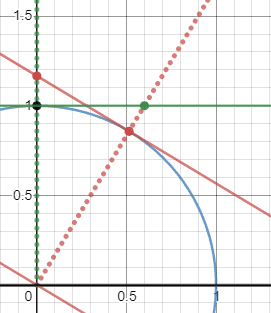# Definition of orthogonality in Minkowski spacetime

• B
• lindberg

#### lindberg

TL;DR Summary
What does it mean for time to be orthogonal on space in Minkowski spacetime?
I have read that non-inertial frames are those, where time is not orthogonal on space. Does it just mean that the speed of light is not isotropic there or does it mean anything else? How can I picture more easily this concept (for space orthogonality I just imagine perpendicularity of one axis on the other, how is it different for time?)
Thanks.

Summary: What does it mean for time to be orthogonal on space in Minkowski spacetime?

I have read that non-inertial frames are those, where time is not orthogonal on space.
Where? It is generally not correct.

•vanhees71
Here is just a brief definition from wiki:So what happens in non-inertial frames? Why are they non-orthogonal?
What is the definition for that?
Here is where I got the idea of non-orthogonality:He goes on to say:
"In general one can conclude that non-invariance and degree of anisotropy for the local, physically measurable speed of light are directly dependent on the slope of the time axis relative to the 3D space axes. All 6 frames for which time is orthogonal to space have isotropic light speed equal to c. All NTO frames have anisotropic light speed not equal to c."

https://arxiv.org/pdf/gr-qc/0005121.pdf
Thanks.

How can I picture more easily this concept (for space orthogonality I just imagine perpendicularity of one axis on the other, how is it different for time?)
Thanks.
And to answer this: It is not different from spatial perpendicularity. The only difference is that perpendicularity is determined by the Minkowski metric and not a Euclidean metric.

•Why are they non-orthogonal?
As i already said, this is not correct. There are accelerated frames where the coordinate time is orthogonal to the spatial directions.

The wiki page is also not saying that accelerated frames are non-orthogonal. It is saying that you can construct an orthogonal basis in an inertial frame. Those are very different statements.

To be blunt: From your posts you do not seem to have mastered the basics of special relativity in inertial frames. I would strongly suggest you obtain that mastery before delving into accelerated frames or your understanding will suffer tremendously.

•...and the "Minowski metric" is not a metric, because it's not positive definite. It's a "fundamental bilinear form" inducing a pseudo-metric rather than a metric. That's why you have "null vectors" ("lightlike vectors") in Minkowski space, i.e., ##x \cdot x=0## but ##x \neq 0##.

•David Lewis, dextercioby, lindberg and 1 other person
I would say an inertial frame is one in which objects moving inertially (i.e., have zero proper acceleration) have zero coordinate acceleration. This definition includes some coordinate systems which have non-orthogonal time axes.

Switching to such a coordinate system makes a lot of things messy, basically because almost all physics requires you at some point to calculate ##\frac d{d t}## at some point. In a non-orthogonal system rate of change of ##t## depends on your rate of change of ##x## as well, so you have a lot of extra derivatives in your maths to handle this. This is why velocities (including that of light) become non-isotropic - because ##\frac{\partial x}{\partial t}## is involved in your definition of velocity and it can be positive, negative, or zero depending on which direction you go.

Non-inertial systems may or may not have time axes orthogonal to their spatial planes. Inertiality or lack thereof of your coordinate system is not generally the same issue as orthogonality.

•vanhees71 and lindberg
To address the issue in the title of this thread... orthogonality in Minkowski spacetime

Based on
and https://physics.stackexchange.com/a/600504/148184

Physically, given the 4-velocity of an inertial observer,
that observer's sense of space is a "[hyper]plane of simultaneity",
which is
geometrically, determined by the tangent [hyperplane] to the "unit hyperboloid" at the tip of the 4-velocity.
This defines "orthogonality" or "perpendicularity" to the 4-velocity vector.

More details (based on my stackexchange answer):
The "lines of simultaneity (i.e, equal-assignments-of-t)" are determined by the tangents to the "circles" [in the respective geometries], which is a hyperbola in Special Relativity (arising from the invariance of the speed of light).

The "circle" is determined by the set of all observer "tick-1"s.
The "circle" defines perpendicularity: the tangent is perpendicular to the radius...
"the Space[line] is Perpendicular to the Time[line] (worldline)", as Minkowski described in his "Space and Time".

From Minkowski's "Space and Time"...

We decompose any vector, such as that from O to x, y, z, t into four components x, y, z, t. If the directions of two vectors are, respectively, that of a radius vector OR from O to one of the surfaces ##\mp F=1##, and that of a tangent RS at the point R on the same surface, the vectors are called normal to each other. Accordingly,

$$c^2tt_1 − xx_1 − yy_1 − zz_1 = 0$$
is the condition for the vectors with components x, y, z, t and ##x_1##, ##y_1##, ##z_1##, ##t_1## to be normal to each other.

See this definition used in
https://www.desmos.com/calculator/r4eij6f9vw (robphy's spacetime diagrammer v.3)
https://www.desmos.com/calculator/kv8szi3ic8 (robphy's spacetime diagrammer for relativity v.8d-2020)

First, vary the ##v_1## and ##v_2## sliders to get a feel for "tangency".

For the E-slider = +1, we have Minkowski spacetime
(on your way to E= -1 for Euclidean space, note the E=0 case is the Galilean spacetime)

Here is E = +1 (Minkowski)E= -1 (Euclidean)Further details: https://physics.stackexchange.com/a/638018/148184

•dextercioby, Sagittarius A-Star, vanhees71 and 1 other person
@Orodruin I think you are right, I have delved into rotating and accelerated frames before being completely clear on inertial ones. Would you recommend some resources for beginners to understand the standard Minkowski geometry in inertial frames?
Thank you.

@Orodruin I think you are right, I have delved into rotating and accelerated frames before being completely clear on inertial ones. Would you recommend some resources for beginners to understand the standard Minkowski geometry in inertial frames?
Thank you.
Here's my try to explain it:

https://itp.uni-frankfurt.de/~hees/pf-faq/srt.pdf

•lindberg
@Orodruin I think you are right, I have delved into rotating and accelerated frames before being completely clear on inertial ones. Would you recommend some resources for beginners to understand the standard Minkowski geometry in inertial frames?
Thank you.
My first recommendation is Taylor and Wheeler’s “Spacetime Physics”

•••vanhees71
My first recommendation is Taylor and Wheeler’s “Spacetime Physics”
First edition, if you can find it.

•lindberg, robphy and vanhees71
First edition, if you can find it.
The first edition… with the worked problems.

"Spacetime Physics" (1966 Maroon cover) by Taylor and Wheeler.
There is a version with about 210 pages and one with 270 pages.
The 270-page version has solutions to problems. (Parts are here on Taylor's website: https://www.eftaylor.com/download.html#special_relativity .
Note: The Second edition sadly does not use rapidity.)

Last edited:
•dextercioby
I'm sorry to take up space, but I'm not sure if this deserves its own thread. Do you think it does?
No, you just need to stop being sloppy and cluttering the thread with incorrect statements.

why are you so angry, anyway?
I'm not angry, I'm doing my job as a moderator. Part of my job is to manage the signal to noise ratio of the forums. Cluttering threads with repeated incorrect statements is noise. Stop doing it.

I didn't come up with some "theory," I'm just pointing out an attribute we observe in the interval metric equation -- specifically, that elapsed time "acts like" negative distance.
Which is wrong, as you have been repeatedly told. You have now been banned from further posting in this thread.